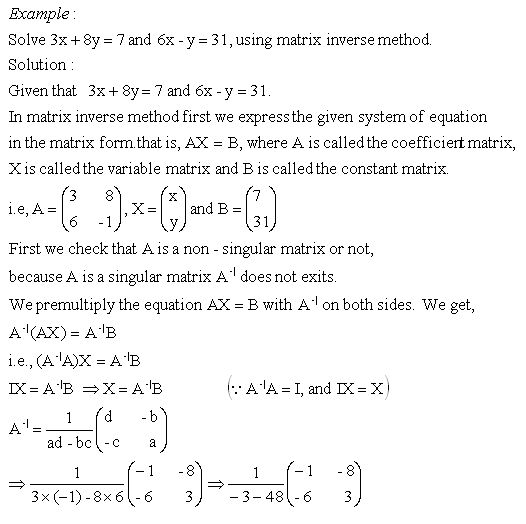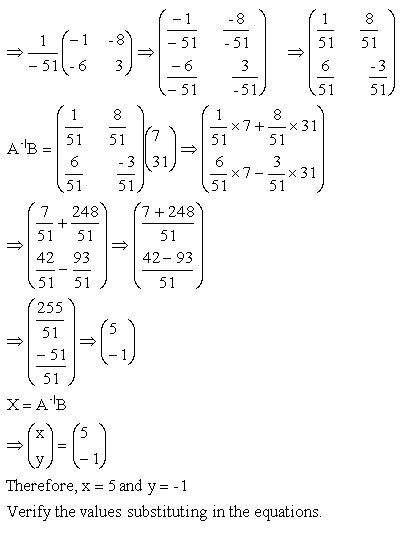Name: ___________________Date:___________________

kwizNET Subscribers, please login to turn off the Ads!
 Email us to get an instant 20% discount on highly effective K-12 Math & English kwizNET Programs!

### High School Mathematics - 26.6 Solving Linear Equations Using Inverse MethodDirections: Solve the following probelms using above method. Also write at least five examples of your own.
 Q 1: Solve, x + 3y = 10 and 3x + 2y = 9.x = 2 and y = 3x = 1 and y = 2x = 4 and y = 3x = 1 and y = 3 Q 2: Solve, x + 2y = 10 and 3x + 4y = 22.x = 4 and y = 2x = 2 and y = 4x = 3 and y = 4x = 2 and y = 3 Q 3: Solve, 3x + 2y = 27 and x + y = 10.x = 6 and y = 4x = 7 and y = 3x = 4 and y = 6x = 8 and y = 2 Q 4: Solve, 5x + 2y = 24 and x + 3y = 10.x = 3 and y = 2x = 4 and y = 3x = 3 and y = 1x = 4 and y = 2 Q 5: Solve, x + 2y = 8 and 2x + 3y = 13.x = 2 and y = 5x = 2 and y = 3x = 2 and y = 2x = 3 and y = 2 Q 6: Solve, x + 3y = 7 and 3x + 4y = 11.x = 2 and y = 1x = 2 and y = 2x = 1 and y = 2x = 1 and y = 3 Question 7: This question is available to subscribers only! Question 8: This question is available to subscribers only!

#### Subscription to kwizNET Learning System costs less than \$1 per month & offers the following benefits:

• Unrestricted access to grade appropriate lessons, quizzes, & printable worksheets
• Instant scoring of online quizzes
• Progress tracking and award certificates to keep your student motivated
• Unlimited practice with auto-generated 'WIZ MATH' quizzes
• Child-friendly website with no advertisements

© 2003-2007 kwizNET Learning System LLC. All rights reserved. This material may not be reproduced, displayed, modified or distributed without the express prior written permission of the copyright holder. For permission, contact info@kwizNET.com
For unlimited printable worksheets & more, go to http://www.kwizNET.com.